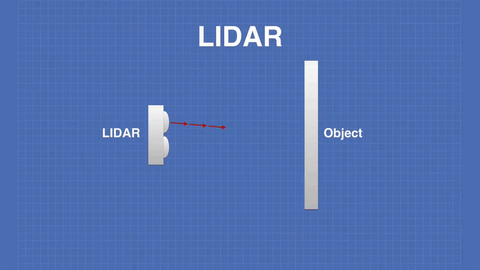# What Is LiDAR and How Does It Work?

Light Detection and Ranging (LiDAR) is an extremely popular method of mapping environments, it's most commonly used in autonomous vehicles, military applications, surveying and robotics. Typically LiDAR uses a laser with a wavelength between 600-1000nm, these wavelengths are used as they do not harm the eye when looked at.

So how does it work?

In the most simple terms, LIDAR works by emitting a pulse of light towards an object and measuring the time it takes for the light to bounce off and return.This time is then used in the Distance-Speed-Time formula where speed is the known speed of light.

Speed = Distance / Time -> Distance = (Speed x Time) / 2

The distance calculated will be for the return trip of the laser pulse so in order to find the distance to the detected object it is divided by 2. There are a number of variations on LiDAR but they all use this principle of a pulse of light being bounced off of an object.